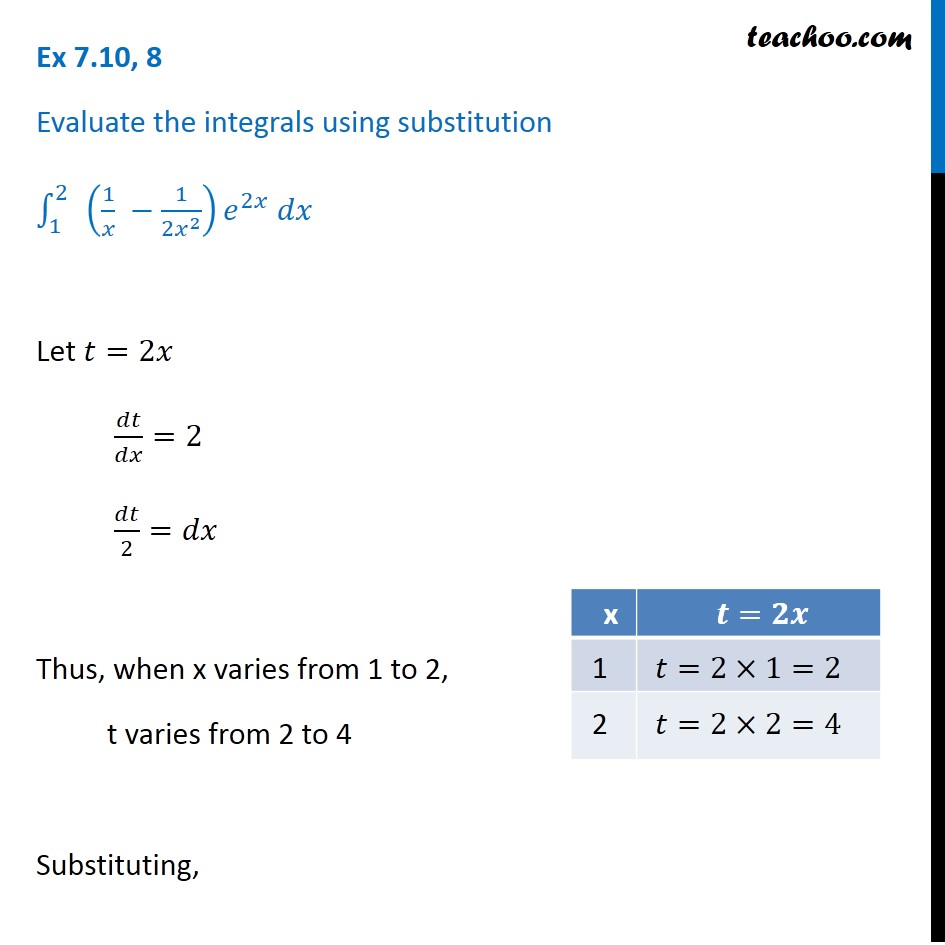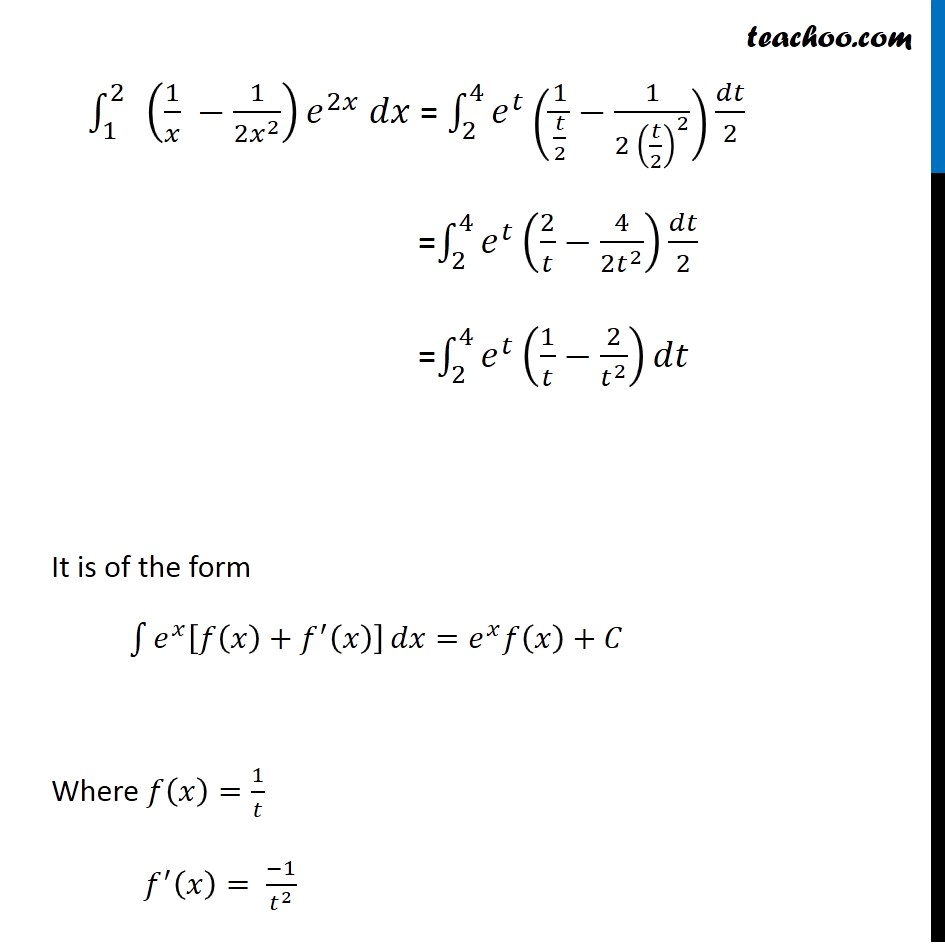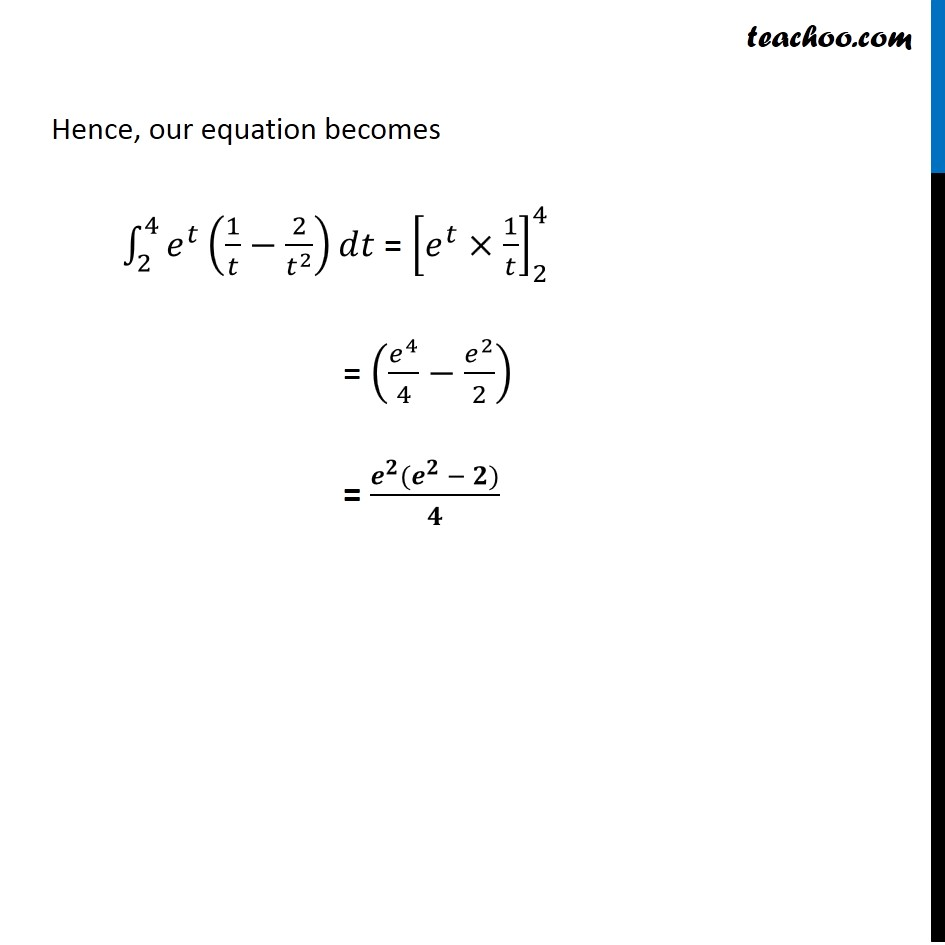Integration Full Chapter Explained - Integration Class 12 - Everything you need1. Chapter 7 Class 12 Integrals
2. Concept wise
3. Definite Integration - By Formulae

Transcript

Ex 7.10, 8 Evaluate the integrals using substitution ∫_1^(2 )▒〖 (1/𝑥 −1/(2𝑥^2 )) 〗 𝑒^2𝑥 𝑑𝑥 Let 𝑡=2𝑥 𝑑𝑡/𝑑𝑥=2 𝑑𝑡/2=𝑑𝑥 Thus, when x varies from 1 to 2, t varies from 2 to 4 Substituting, ∫_1^(2 )▒〖 (1/𝑥 −1/(2𝑥^2 )) 〗 𝑒^2𝑥 𝑑𝑥 = ∫_2^4▒〖𝑒^𝑡 (1/(𝑡/2)−1/(2〖 (𝑡/2)〗^2 )) 〗 𝑑𝑡/2 =∫_2^4▒〖𝑒^𝑡 (2/𝑡−4/(2𝑡^2 )) 〗 𝑑𝑡/2 =∫_2^4▒〖𝑒^𝑡 (1/𝑡−2/𝑡^2 ) 〗 𝑑𝑡 It is of the form ∫1▒〖𝑒^𝑥 [𝑓(𝑥)+𝑓^′ (𝑥)] 〗 𝑑𝑥=𝑒^𝑥 𝑓(𝑥)+𝐶 Where 𝑓(𝑥)=1/𝑡 𝑓^′ (𝑥)= (−1)/𝑡^2 Hence, our equation becomes ∫_2^4▒〖𝑒^𝑡 (1/𝑡−2/𝑡^2 ) 〗 𝑑𝑡 = [𝑒^𝑡×1/𝑡]_2^4 = (𝑒^4/4−𝑒^2/2) = (𝒆^𝟐 (𝒆^𝟐 − 𝟐))/𝟒

Definite Integration - By Formulae

About the AuthorDavneet Singh
Davneet Singh is a graduate from Indian Institute of Technology, Kanpur. He has been teaching from the past 9 years. He provides courses for Maths and Science at Teachoo.